Technical Article

# How to Design a Precision Current Pump with Op-Amps

October 16, 2020 by Robert Keim

## This article discusses a voltage-controlled current-source circuit that requires only two op-amps and a handful of resistors.

In circuit theory, voltage sources and current sources are equally ideal and equally easy to implement. You just draw a circle, and then you add plus and minus signs for voltage or an arrow for current. Now you have a circuit element that generates a specified voltage under all conditions or that drives a specified current under all conditions.

In real life, sources are not ideal, and furthermore, approximating a theoretical voltage source is significantly easier than approximating a theoretical current source. Voltage sources are as simple as a battery, a zener diode, or a resistive voltage divider combined with a buffer.

Current sources, on the other hand, usually require some clever circuit design and greater attention to operational details.

### Current-Source Architectures

There are various ways to design a current source. Before we look at a two-op-amp topology, let’s briefly review some other options. You can learn more about all these topics by clicking on the respective links.

One interesting approach is to use a voltage regulator as a current regulator: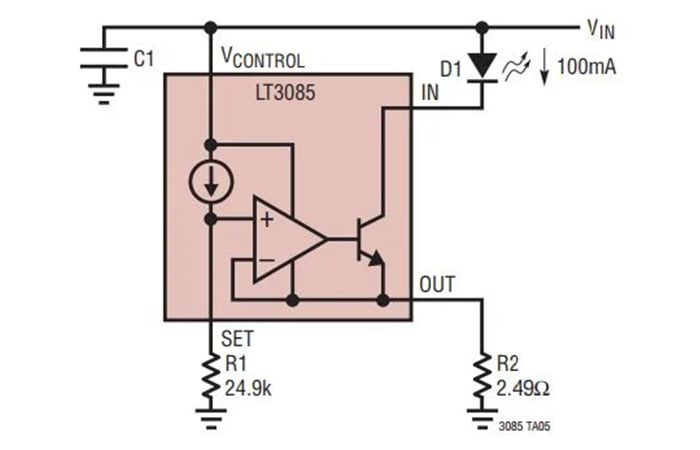##### Application diagram of the LT3085. Image used courtesy of Linear Devices (Analog Devices)

Another option is an amplifier-based circuit that I discussed in a previous article on how to design a simple, voltage-controlled, bidirectional current source. An amplifier-based circuit is vaguely reminiscent of the two-op-amp topology, but one of the amplifiers is an instrumentation amp rather than an op-amp.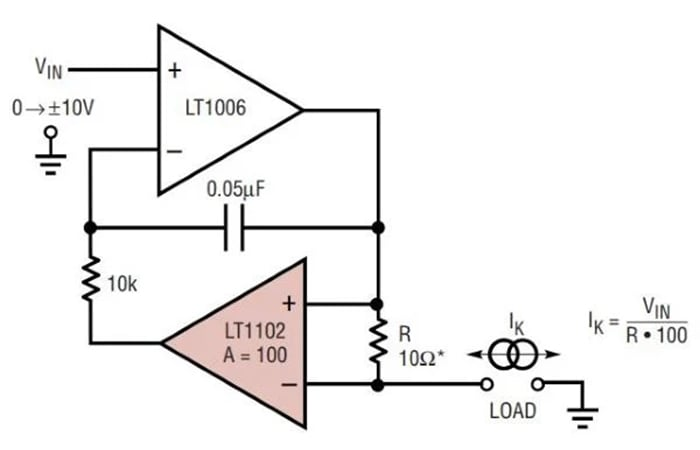##### Diagram of a voltage-programmable current source. Image used courtesy of Linear Devices (Analog Devices)

Finally, we have the Howland current pump, which was thoroughly analyzed in an AAC article written by Dr. Sergio Franco.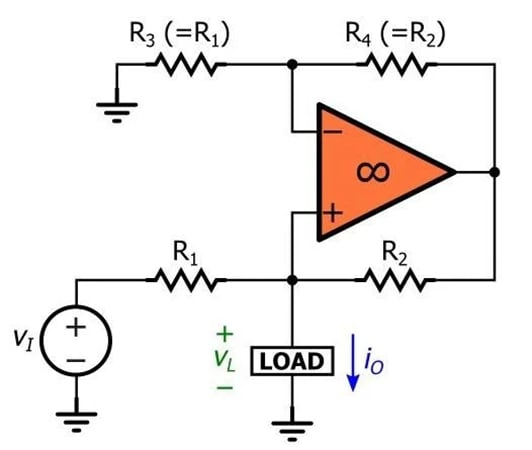### The Two-Op-Amp Topology

I found this circuit, which is described as a “precision current pump,” in an old app note from Analog Devices. It produces a bidirectional output current that’s directly proportional to an input voltage.

Here’s the original circuit diagram: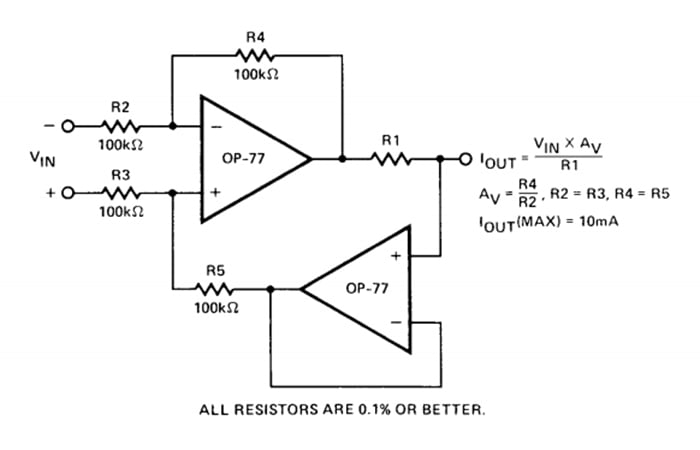##### Diagram of a precision current pump. Image used courtesy of Analog Devices

There are a few things that I like about this circuit. First, only two types of components are needed: op-amps and resistors.

Second, the op-amps have the same part number. It’s true that this circuit uses two op-amps whereas the Howland pump uses only one, but the fact that both op-amps can be the same exact part is advantageous because you can use a dual-op-amp IC package and thereby minimize whatever additional cost or board space is required for the second op-amp.

Third, four of the five resistors (R2, R3, R4, R5) can have the same value, and then the voltage-to-current gain is controlled by one resistor (R1). The value of R2–R5 isn’t critical, so you can adapt the circuit to components that you already have in the lab or to an existing BOM. Keep in mind, though, that higher-precision resistors will produce a higher-precision current source.

Fourth, the input voltage is differential. This gives you some flexibility in how you provide the control voltage, and it allows you to take advantage of the circuit’s bidirectional output-current capability without the need to generate a control voltage that extends below ground.

### Basic Operation of a Two-Op-Amp Current Source

We’ll use an LTspice implementation to help us analyze the two-op-amp current source.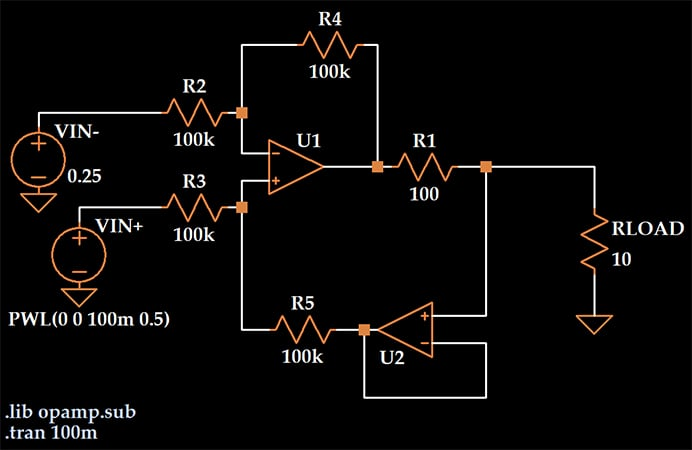Here I’m using the LTspice “ideal single-pole operational amplifier.” I initially tried it with the OP-77, but the simulation didn’t run properly. There might have been an issue with the OP-77 macromodel, because I have another version of the circuit that uses the LT1001A op-amp and it simulates correctly.

Constant-current-source circuits generally rely on some type of feedback that causes a voltage source to produce a specified current regardless of the load resistance. (You can see a straightforward example of this in the voltage-controlled LED driver that I designed for a color-sensor project.)

In the two-op-amp current pump, U1 amplifies the differential control voltage, and U2 is configured as a voltage follower that senses the voltage across the load and feeds it back to the input stage.

The voltage-source configuration shown above produces a differential input voltage that varies from +250 mV to –250 mV. According to the equation provided in the app note, the output current should vary from 2.5 mA to –2.5 mA, since AV = 1 and R1 = 100 Ω, and this is exactly what we observe: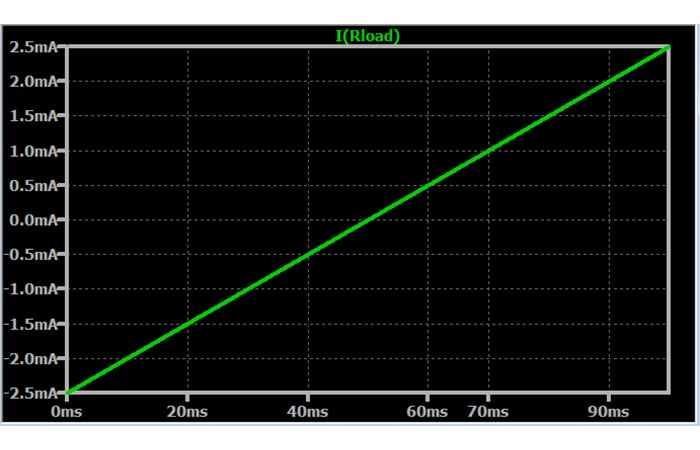One thing that you need to watch out for with this circuit is the U1 output voltage. All of the load current comes from U1. If we neglect the very small currents that flow through the feedback resistor R4 and into the positive input terminal of U2, the voltage at U1’s output terminal will be equal to IOUT multiplied by the sum of the load resistance and the resistance of R1.

$V_{OUT,U1}\approx \left(R_{LOAD}+R1\right)I_{OUT}$

This voltage can easily exceed what the op-amp’s output stage can actually generate, especially if you’re using ±3 V or ±5 V rails rather than the ±12 V or ±15 V analog supply voltages that were, I believe, more common in the past.

Because of this limitation, I would say that the two-op-amp current pump is a good choice for applications with low load resistance and/or small output currents.

### Conclusion

We’ve taken a quick look at a bidirectional current-source circuit that has reasonable BOM requirements and incorporates a differential control-voltage input stage. In the next article, we’ll use LTspice to analyze the circuit’s performance in greater detail.